# Evaluating Formative Measurement Model - Step 3: Indicator Weights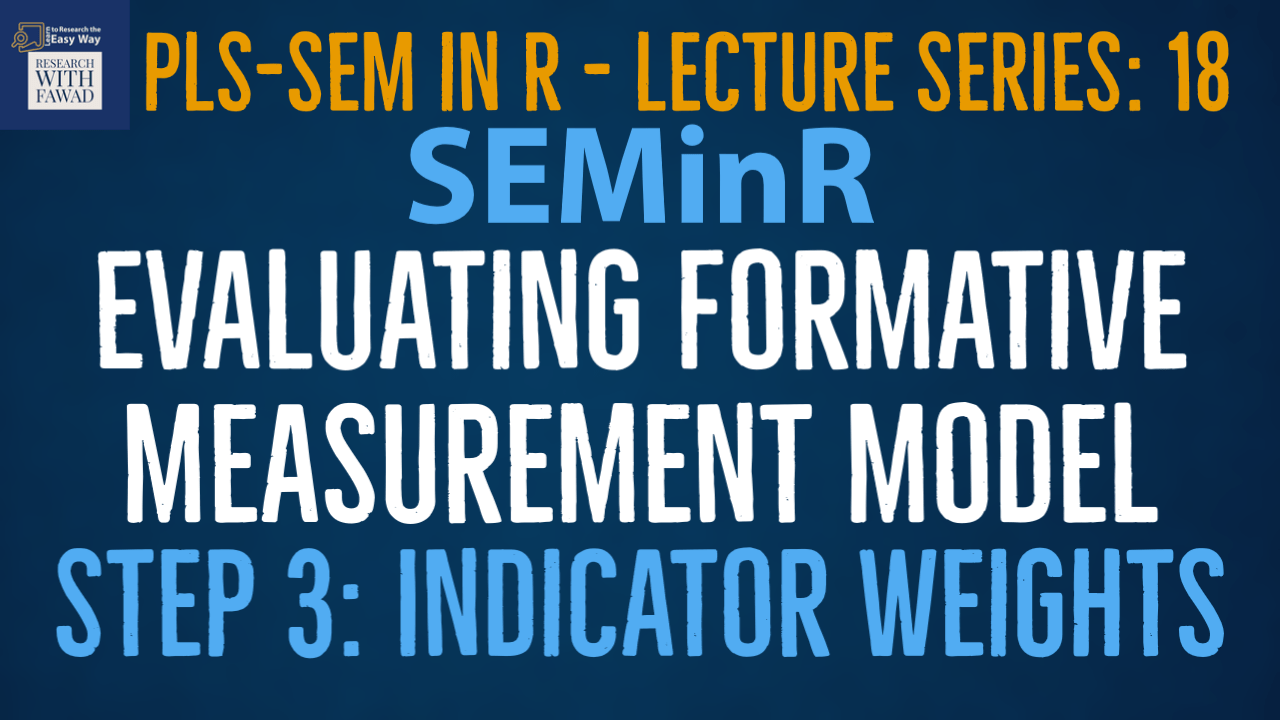### SEMinR Lecture Series

This session is focused on step 3 of the formative measurement model assessment. The tutorial will guide on how to assess the indicator weights using SEMinR.

## Evaluating Formative Measurement Model

• PLS-SEM is the preferred approach when formatively specified constructs are included in the PLS path model (Hair, Risher, Sarstedt, & Ringle, 2019).
• In this part of the series, I discuss the key steps for evaluating formative measurement models (Fig.). Relevant criteria include the assessment of Convergent validity,indicator collinearity, and Statistical significance and relevance of the indicator weights.
• Next, I will introduce key criteria and their thresholds and illustrate their use with an example.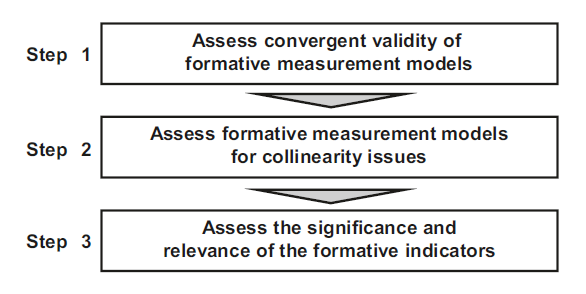## The Example

• The proposed model has three constructs (Vision, Development, and Reward) measured formatively that impact a reflectively measured construct (Collaborative Culture).
• The three constructs are formative constructs, estimated with mode_B, while Collaborative Culture is reflective construct, estimated with mode_A.
• The weights parameter of the composite() function is set by default to mode_A. Thus, when no weights are specified, the construct is estimated as being reflective.
• Alternatively, we can explicitly specify the mode_A setting for reflectively measured constructs or the mode_B setting for formatively measured constructs.
• Once the model is set up, we use the estimate_pls() function to estimate the model, this time specifying the measurement_model and structural_model.
• Finally, we apply the summary() function to the estimated SEMinR model object simple_model and store the output in the summary_simple object.

## Step 3: Indicator Weights

• Next, analyze the indicator weights for their significance and relevance.
• First, consider the significance of the indicator weights by means of bootstrapping. To run the bootstrapping procedure using the bootstrap_model() function.
• The first parameter (i.e., seminr_model) allows specifying the model on which we apply bootstrapping. The second parameter nboot allows us to select the number of bootstrap samples to use. Per default, we should use 10,000 bootstrap samples (Streukens & Leroi-Werelds, 2016).
• Since using such a great number of samples requires much computational time, you may choose a smaller number of samples (e.g., 1,000) for the initial model estimation.
• For the final result reporting, however, we should use the recommended number of 10,000 bootstrap samples.
• The cores parameter enables us to use multiple cores of your computer’s central processing unit (CPU). Recommended is using this option since it makes bootstrapping much faster.
• As you might not know the number of cores in your device, recommended is using the parallel::detectCores() function to automatically detect the number of cores and use the maximum cores available.
• By default, cores will be set to the maximum value and as such, if you do not specify this parameter, your bootstrap will default to using the maximum computing power of your CPU.
• Finally, seed allows reproducing the results of a specific bootstrap run while maintaining the random nature of the process.
• Assign the output of the bootstrap_model() function to the summary_boot object.
•
• Finally, we need to run the summary() function on the summary_boot object and set the alpha parameter. The alpha parameter allows selecting the significance level (the default is 0.05) for two-tailed testing. When testing indicator weights, we follow general convention and apply two-tailed testing at a significance level of 5%.
•  Following is the complete code from the previous sessions (Step 1: Convergent Validity and Step 2: Collinearity Diagnostics on evaluating formative model evaluation.
• Line 27-28 is for Step 3, Assessing Indicator Weights.

## The Code

``````library(seminr)
# Create measurement model
simple_mm <- constructs(
composite("Vision", multi_items("VIS", 1:4), weights = mode_B),
composite("Development", multi_items("DEV", 1:7), weights = mode_B),
composite("Rewards", multi_items("RW",1:4), weights = mode_B),
composite("Collaborative Culture", multi_items("CC", 1:6)))
# Create structural model
simple_sm <- relationships(
paths(from = c("Vision","Development","Rewards"), to = "Collaborative Culture"))
# Estimate the model
simple_model <- estimate_pls(data = datas,
measurement_model = simple_mm,
structural_model = simple_sm,
missing = mean_replacement,
missing_value = "-99")
# Summarize the model results
summary_simple <- summary(simple_model)
# Bootstrap the PLS Estimated Model
boot_model <- bootstrap_model(seminr_model = simple_model, nboot = 1000, cores = parallel::detectCores(), seed = 123)
# Store the summary of the bootstrapped model
# alpha sets the specified level for significance, i.e. 0.05
summary_boot <- summary(boot_model, alpha = 0.05)
# Inspect the bootstrapping results for indicator weights
summary_boot\$bootstrapped_weights``````

### Step 3: Statistical Significance and Relevance of the Indicator Weights

• The third step in assessing formatively measured constructs is examining the statistical significance and relevance (i.e., size) of the indicator weights.
• The indicator weights result from regressing each formatively measured construct on its associated indicators. As such, they represent each indicator’s relative importance for forming the construct.
• Significance testing of the indicator weights relies on the bootstrapping procedure.
• The bootstrapping procedure yields t-values for the indicator weights (and other model parameters).
• Assuming a significance level of 5%, a t-value above 1.96 (two tailed test) suggests that the indicator weight is statistically significant. The critical values for significance levels of 1% (α = 0.01) and 10% (α = 0.10) probability of error are 2.576 and 1.645 (two tailed), respectively.
• Inspect the summary_boot\$bootstrapped_weights.
• Figure shows t-values for the measurement model relationships
• Note that bootstrapped values are generated for all measurement model weights, but we only consider the indicators of the formative constructs.
• Recall that the critical values for significance levels of 1% (α = 0.01), 5% (α = 0.05), and 10% (α = 0.10) probability of error are 2.576, 1.960, and 1.645 (two tailed), respectively.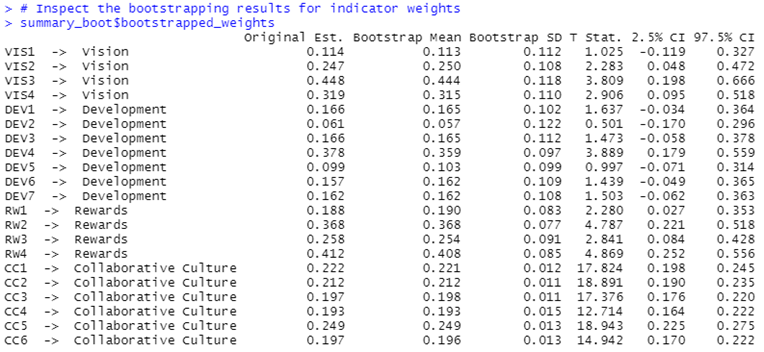• Confidence intervals are an alternative way to test for the significance of indicator weights. They represent the range within which the population parameter will fall assuming a certain level of confidence (e.g., 95%).
• If a confidence interval does not include the value zero, the weight can be considered statistically significant, and the indicator can be retained.
• On the contrary, if the confidence interval of an indicator weight includes zero, this indicates the weight is not statistically significant (assuming the given significance level, e.g., 5%). In such a situation, the indicator should be considered for removal from the measurement model.
• However, if an indicator weight is not significant, it is not necessarily interpreted as evidence of poor measurement model quality.
• We recommend you also consider the absolute contribution of a formative indicator to the construct (Cenfetelli & Bassellier, 2009), which is determined by the formative indicator’s loading.
• At a minimum, a formative indicator’s loading should be statistically significant. Indicator loadings of 0.5 and higher suggest the indicator makes a sufficient absolute contribution to forming the construct.
• The lower boundary of the 95% confidence interval (2.5% CI) is displayed in the second-to-last column, whereas the upper boundary of the confidence interval (97.5% CI) is shown in the last column.
• We can readily use these confidence intervals for significance testing. if a confidence interval for an estimated coefficient does not include zero, the hypothesis that w1 equals zero is rejected, and we assume a significant effect.
• Looking at the significance levels, we find that all formative indicators are significant at a 5% level.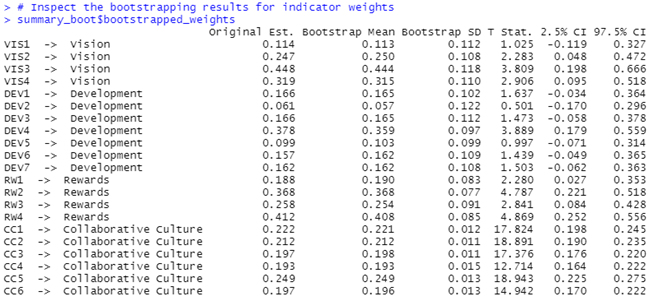• Next, to assess these indicators’ absolute importance, we examine the indicator loadings by running

• The output in Fig. (column: Original Est.) shows that the indicator loadings over .70 for all the formative indicators. Furthermore, results from bootstrapping show that the t-values of the formative indicator loadings are clearly above 2.576, suggesting that all indicator loadings are significant even at a level of 1% (Fig).
• We retain all indicators in the formatively measured constructs, even though not every indicator weight is significant. The analysis of indicator weights concludes the evaluation of the formative measurement models.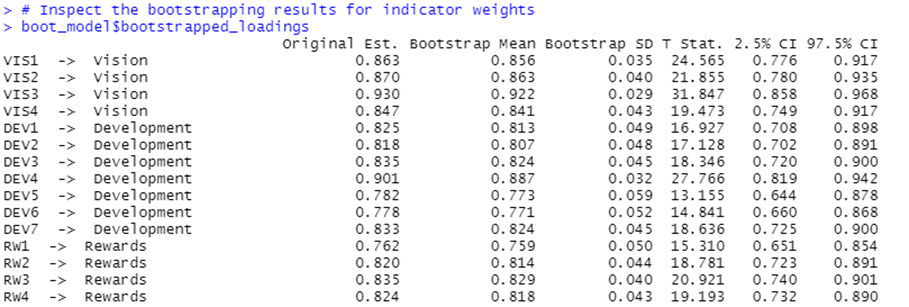## Complete Code

``````library(seminr)
# Create measurement model
simple_mm <- constructs(
composite("Vision", multi_items("VIS", 1:4), weights = mode_B),
composite("Development", multi_items("DEV", 1:7), weights = mode_B),
composite("Rewards", multi_items("RW",1:4), weights = mode_B),
composite("Collaborative Culture", multi_items("CC", 1:6)))
# Create structural model
simple_sm <- relationships(paths(from = c("Vision", "Development", "Rewards"), to = "Collaborative Culture"))
# Estimate the model
simple_model <- estimate_pls(data = datas, measurement_model = simple_mm, structural_model = simple_sm, missing = mean_replacement, missing_value = "-99")
# Summarize the model results
summary_simple <- summary(simple_model)
#Descriptive Stattistics Summary
summary_simple\$descriptives\$statistics
# Iterations to converge
summary_simple\$iterations
# Collinearity analysis
summary_simple\$validity\$vif_items
# Bootstrap the model on the PLS Estimated Model
boot_model <- bootstrap_model(
seminr_model = simple_model,
nboot = 1000, cores = parallel::detectCores(), seed = 123)
# Store the summary of the bootstrapped model
# alpha sets the specified level for significance, i.e. 0.05
summary_boot <- summary(boot_model, alpha = 0.05)
# Inspect the bootstrapping results for indicator weights
summary_boot\$bootstrapped_weights

## Reference

Hair Jr, J. F., Hult, G. T. M., Ringle, C. M., Sarstedt, M., Danks, N. P., & Ray, S. (2021). Partial Least Squares Structural Equation Modeling (PLS-SEM) Using R: A Workbook.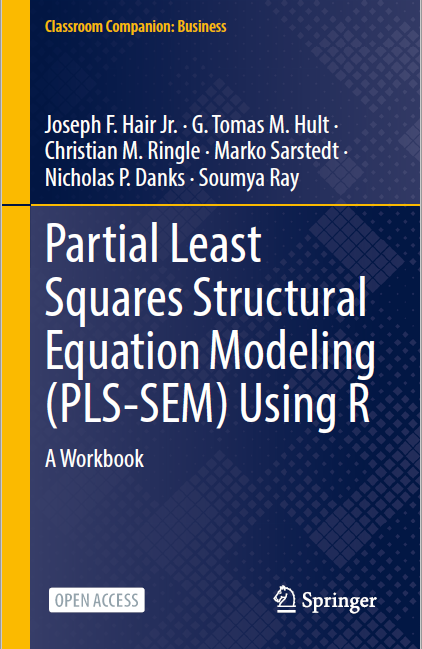The tutorials on SEMinR are based on the mentioned book. The book is open source and available for download under this link.